# Go Math Grade 3 Chapter 3 Answer Key Pdf Understand Multiplication

Lesson 1: Count Equal Groups

Lesson 2: Algebra • Relate Addition and Multiplication

Lesson 3: Skip Count on a Number Line

Mid-Chapter Checkpoint

Lesson 4: Problem Solving • Model Multiplication

Lesson 5: Model with Arrays

Lesson 6: Algebra • Commutative Property of Multiplication

Lesson 7: Algebra • Multiply with 1 and 0

Chapter 3 Review/Test

### Count Equal Groups Page No 143

Draw equal groups. Skip count to find how many.

Question 1.
2 groups of 2 4Explanation:
There are two groups of 2
There are 2 two’s.
We skip count by 2’s. (2, 4)
So, there are 4 in all.

Question 2.
3 groups of 6 ________

Explanation:
Draw 6 counters in each group.
There are 3 equal groups.
skip count by six until you say 3 numbers.
There are 3 equal groups with 6 counters in each group.
So, there are 18 counters in all.

Question 3.
5 groups of 3 ________

Explanation:
Draw 3 counters in each group.
There are 5 equal groups.
Now, skip count by 3’s until you say 5 numbers.
There are 5 equal groups with 3 counters in each group.
So, there are 15 counters in all.

Question 4.
4 groups of 5 ________

Explanation:
Draw 5 counters in each group.
There are 4 equal groups.
Skip count by 5’s until you say 4 numbers.
There are 4 equal groups with 5 counters in each group.
So, there are 20 counters in all.

Count equal groups to find how many.

Chapter 3 Understand Multiplication Answer Key Question 5.______ groups of _______ , _______ in all

Explanation:
There are three counters in each group.
There are 4 equal groups.
We skip count by 3’s until you say 4 numbers. (3,6,9,12)
So, there are 4 groups of 3, 12 in all.

Question 6.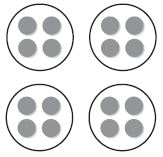______ groups of _______ , _______ in all

Explanation:
There are 4 counters in each group
There are 4 equal groups.
We skip count by 4’s until you say 4 numbers. (4,8,12,16)
There are 4 groups of 4, 16 in all

Problem Solving

Question 7.
Marcia puts 2 slices of cheese on each sandwich. She makes 4 cheese sandwiches. How many slices of cheese does Marcia use in all?
_________ slice of cheese

Explanation:
Draw 2 counters (cheese) in each group.
There are 4 equal groups (sandwiches).
We skip count by 2’s until you say 4 numbers (4,8)
There are 8 slices of cheese.

Question 8.
Tomas works in a cafeteria kitchen. He puts 3 cherry tomatoes on each of 5 salads. How many tomatoes does he use?
_________ tomatoes

Explanation:
Draw 3 counters (tomatoes) in each group.
There are 5 equal groups (salads).
We skip count by 3’s until you say 5 numbers (3,6,9,12,15)
There are 15 tomatoes in all.

### Count Equal Groups Lesson Check Page No 144

Question 1.
Jen makes 3 bracelets. Each bracelet has 3 beads. How many beads does Jen use?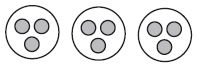Options:
a. 12
b. 9
c. 6
d. 3

Explanation:
There are 3 beads in each group.
There are 3 equal groups (bracelets).
Now, skip count by 3’s until you say three numbers (3,6,9)
Jen use a total of 9 beads.

Question 2.
Ian has 5 cards to mail. Each card needs 2 stamps. How many stamps does Ian need?Options:
a. 2
b. 5
c. 10
d. 15

Explanation:
There are 5 equal groups (cards) with two counters (stamps) in each group.
Now, skip count by 2’s until you say five numbers (2,4,6,8,10)
Ian need a total of 1o stamps.

Spiral Review

Question 3.
There were 384 people at a play on Friday night. There were 512 people at the play on Saturday night. Which is the best estimate of the total number of people who attended the play on both nights?
Options:
a. 900
b. 800
c. 700
d. 500

Explanation:
Step 1: Round each number to nearest hundred.
384 —-> 400
512  —-> 500
Step 2: Add the rounded numbers
400 + 500 = 900
The best estimate of the total number of people who attended the play on both nights are 900.

Question 4.
Walking the Dog Pet Store has 438 leashes in stock. They sell 79 leashes during a one-day sale. How many leashes are left in stock after the sale?
Options:
a. 459
b. 441
c. 369
d. 359

Explanation: Use place value to subtract
Subtract 438 – 79
Estimate 450 – 100 =

Step 1
Subtract the ones.
8 < 9, so regroup.
3 tens 8 ones = 2 tens ____ onesOn subtracting the ones place decimals, we get 9

Step 2
Subtract the tens.
2 < 3, so regroup.
4 hundreds 2 tens = 3 hundreds _____ tensOn subtracting the ones and tens place decimals, we get 59

Step 3:
Subtract the hundredsQuestion 5.
The Lakeside Tour bus traveled 490 miles on Saturday and 225 miles on Sunday. About how many more miles did it travel on Saturday?
Options:
a. 500 miles
b. 400 miles
c. 300 miles
d. 100 miles

Explanation:
Use compatible numbers
Step 1: Round each number to nearest hundred.
490 —-> 500
225 —-> 200
Step 2: Subtract the rounded numbers.

(i) Working each column from right to left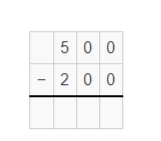(ii) 0 minus 0 is 0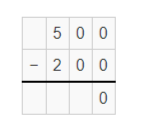(iii) 0 minus 0 is 0(iv) 5 minus 2 is 3Go Math Grade 3 Chapter 3 Question 6.
During one week at Jackson School, 210 students buy milk and 196 students buy juice. How many drinks are sold that week?
Options:
a. 496
b. 406
c. 396
d. 306

Explanation:
Estimate 200 + 200

Step 1: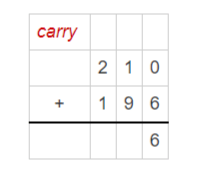Step 2:Step 3:
Add the hundreds. Regroup the tens as hundreds.### Relate Addition and Multiplication Page No 149

Draw a quick picture to show the equal groups. Then write related addition and multiplication sentences.

Question 1.
3 groups of 55 + 5 + 5 = 15
3 × 5 = 15

Explanation:
There are 5 counters in each group.
There are 3 groups.
5 + 5 + 5 = 15
Multiplication Sentence:
Since there are same number of counters in each circle, you can multiply to find how many in all.
Use multiplication method to find the total counters in equal groups.
Factor x Factor = Product
5    x     3       =  15Question 2.
3 groups of 4
_____ + _____ + _____ = ______
_____ × _____ = ______

Answer: 4 + 4 + 4  = 12
3 x 4 = 12

Draw 4 counters in each group.
There are total 3 groups.
Now, the addition sentence is 4 + 4 + 4 = 12

Multiplication sentence
Draw 4 counters in each circle or group.
Since there are same number of counters in each group, multiply groups and counters to find how many there are altogether.
3 x 4 = 12
factor x factor = product

Question 3.
4 groups of 3
_____ + _____ + _____ + _____ = ______
_____ × _____ = ______

Answer: 3 + 3 + 3 + 3 = 12
4 x 3 = 12

Explanation:
Draw 3 counters in each group.
There are total 4 groups.
Now, the addition sentence is 3 + 3 + 3 + 3 = 12

Multiplication sentence
Draw 3 counters in each circle or group.
Since there are same number of counters in each group, multiply counters and groups to find how many there are altogether.
4 x 3 = 12
factor x factor = product

5 groups of 2
_____ + _____ + _____ + _____ + _____ = ______
_____ × _____ = ______

Answer: 2 + 2 + 2 + 2 + 2 = 10
5 x 2 = 10

Explanation:
Draw 2 counters in each group.
There are total 5 groups.
Now, the addition sentence is 2 + 2 + 2 + 2 + 2 = 12

Multiplication sentence
Draw 2 counters in each circle or group.
Since there are same number of counters in each group, multiply counters and groups to find how many there are altogether.
5 x 2 = 12
factor x factor = product

Complete. Write a multiplication sentence.

Question 5.
7 + 7 + 7 = _____
_____ × _____ = ______

Answer: 7 + 7 + 7 = 21
3 x 7 = 21

Explanation:
7 + 7 + 7 = 21
This is addition sentence, from this we can find the total no of groups and counters.
From above sentence, we can tell that there are 3 groups and 7 counters in each group.
Multiplication Sentence
There are 3 groups and 7 counters in each group.
Since there are same number of counters in each group, multiply counters and groups to find how many there are altogether.
3  x  7 =  21

Question 6.
3 + 3 + 3 = _____
_____ × _____ = ______

Answer: 3 + 3 + 3 = 9
3 x 3 = 9

Explanation:
3 + 3 + 3 = 9
This is addition sentence, from this we can find the total no of groups and counters.
From above sentence, we can tell that there are 3 groups and 3 counters in each group.
Multiplication Sentence
There are 3 groups and 3 counters in each group.
Since there are same number of counters in each group, multiply counters and groups to find how many there are altogether.
3  x  3 =  9

Problem Solving

Question 7.
There are 6 jars of pickles in a box. Ed has 3 boxes of pickles. How many jars of pickles does he have in all? Write a multiplication sentence to find the answer.
_____ × _____ = ______ jars

Answer: 3 x 6 = 18 jars

Explanation:
Draw 3 boxes as Pickle boxes.
Draw 6 counters in each box to show jars.
Now, find the number of counters (jars).
Since, there are same number of jars in each pickle box, you can multiply to find how many in all.
3 groups of 6 = 3 x 6 = 18

Question 8.
Each day, Jani rides her bike 5 miles. How many miles does Jani ride in all in 4 days? Write a multiplication sentence to find the answer.
_____ × _____ = ______ miles

Answer: 4 x 5 = 20 miles

Explanation:
Draw 4 circles to show 4 days.
Draw 5 counters to show miles.
Now, find the total no of miles ride by Jani in 4 days.
Since, Jani rides same number of miles each day, you can multiply to find how many in all.
4 groups of 5 = 4 x 5 = 20

### Relate Addition and Multiplication Lesson Check Page No 150

Question 1.
Which is another way to show
3 + 3 + 3 + 3 + 3 + 3?
Options:
a. 5 × 3
b. 4 × 3
c. 8 × 3
d. 6 × 3

Explanation:
The given question is in the form of addition sentence. Now, we can find the no of counters and groups using it.
Draw 6 circles to show equal groups.
Then, draw 3 counters in each circle.
When you combine equal groups, you can multiply to find how many in all.
No. of equal groups x no of counters = total no of counters
6 x 3 = 18

Question 2.
Use the model. How many counters are there in all?Options:
a. 8
b. 10
c. 12
d. 14

Explanation:
From the above figure, we can see there are two equal groups.
There are 5 counters in each group.
Now, multiplication sentence to find the number of counters.
No. of equal groups x No. of counters in each group = Total no. of counters
2 x 5 = 10

Spiral Review

Question 3.
A school gave 884 pencils to students on the first day of school. What is 884 rounded to the nearest hundred?
Options:
a. 800
b. 880
c. 890
d. 900

Explanation:
We need to round 884 to nearest hundred.
Now, look at the digit to the right which is 8.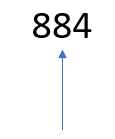8 > 5
So, the hundreds digit increases by one.
Write 9 as the hundreds digit.
Write zeros as the tens and ones digits.
So, 884 rounded to nearest hundred is 900

Question 4.
Find the difference.
6 3 2
– 2 7 4
———
Options:
a. 906
b. 442
c. 358
d. 354

Explanation:
Step-1:
Working each column from right to left.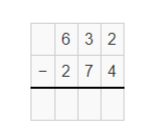Step-2:
Subtract the ones. Since, 2 < 4 , you must regroup.
3 tens 2 ones = 2 tens ______ ones.Step-3:
Subtract the tens. Since 2 < 7, you must regroup.
6 hundreds 2 tens = 5 hundreds ______ ones.Step-4:
Subtract the hundreds.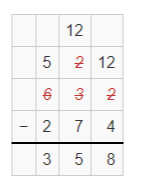Step-5:Question 5.
The line plot below shows how many points Trevor scored in 20 games.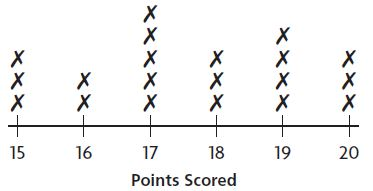In how many games did Trevor score at least 18 points?
Options:
a. 3
b. 5
c. 6
d. 10

Explanation:
The numbers in the line plot shows the points scored by Trevor.
Each in the figure stands for 1 game.
Find 18 points scored on the number line.
In the above line plot, we can see the three s above 18, four s above 19, 3 s above 20.
So, We can say Trevor scored at least 18 points in 10 games.

Question 5.
In how many games did Trevor score 18 points or fewer?
Options:
a. 7
b. 10
c. 13
d. 15

Explanation:
The numbers in the line plot shows the points scored by Trevor.
Each in the figure stands for 1 game.
Find 18 and below 18 points scored on the number line.
In the above line plot, we can see the three s above 18, five s above 17, two s above 16, three s above 15 .
So, We can say Trevor scored at least 18 points in 13 games.

Question 6.
Darrien read 97 pages last week. Evan read 84 pages last week. How many pages in all did the boys read?
Options:
a. 13
b. 171
c. 181
d. 271

Explanation:
Step 1:
Add ones. Regroup the ones as tens and ones.Step 2: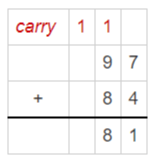Step 3: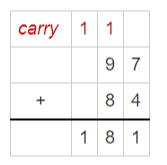### Skip Count on a Number Line Page No 155

Draw jumps on the number line to show equal groups. Find the product.

Question 1.
6 groups of 3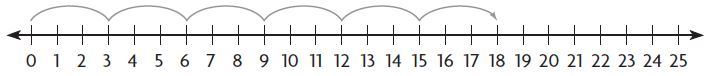6 × 3 = 18

Explanation:
1 jump on the number line is considered as 1 group.
There are 6 jumps on the number line. So, there are 6 groups.
The length of each jump is 3.
Begin at 0. Skip count by 3’s.
Multiply 6 x 3 = 18

Grade 3 Chapter 3 Understand Multiplication Question 2.
3 groups of 5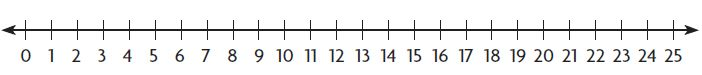3 × 5 = _______

Explanation: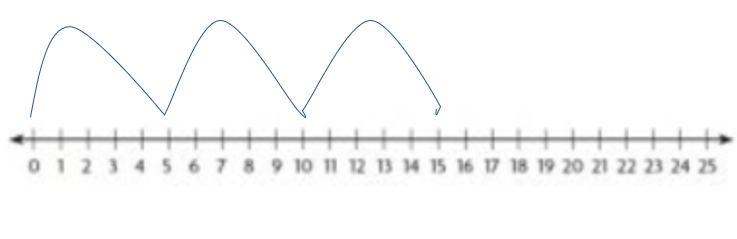1 jump on the number line is considered as 1 group.
There are 5 jumps on the number line. So, there are 5 groups.
The length of each jump is 3.
Begin at 0. Skip count by 3’s.
Multiply 3 x 5 = 15.

Write the multiplication sentence the number line shows.

Question 3.
2 groups of 6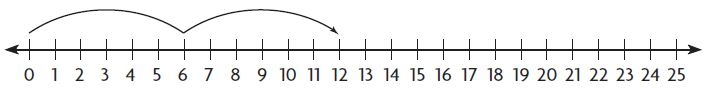______ × _____ = _______

Explanation:
1 jump on the number is considered as 1 group.
There are 2 jumps on a number line. So, there are 2 groups.
The length of each jump is 2.
Begin at 0. Skip count by 6’s.
Multiply 2 x 6 = 12.

Problem Solving

Question 4.
Allie is baking muffins for students in her class. There are 6 muffins in each baking tray. She bakes 5 trays of muffins. How many muffins is she baking in all?
________ muffins

Explanation:
There are 5 baking trays with 6 muffins in each tray.
Consider 1 tray as 1 jump and 1 muffin as length of each jump.
Now, use a number line to find how many muffins allie baked in all.
Begin at 0. Skip count by 6s by drawing jumps on the number line.
No. of jumps made = 5
Length of each jump = 6
Multiply. 5 x 6 = 30
So, Allie bakes 30 muffins in all.

Question 5.
A snack package has 4 cheese sticks. How many cheese sticks are in 4 packages?
________ cheese sticks

Explanation:
There are 4 packages with 4 cheese sticks in each package.
Consider 1 package as 1 jump and 1 cheese stick as length of each jump.
Now, use a number line to find how many cheese sticks are in 4 packages.
Begin at 0. Skip count by 4s by drawing jumps on the number line.
No. of jumps made = 4
Length of each jump = 4
Multiply. 4 x 4 = 16
So, there are 16 cheese sticks in 4 packages.

### Skip Count on a Number Line Lesson Check Page No 156

Question 1.
Louise skip counts by 4 on a number line to find 5 x 4. How many jumps should she draw on the number line?
Options:
a. 3
b. 4
c. 5
d. 9

Explanation:
Given no. of skip counts on a number line = 4
Product = no. of jumps x length of each jump
Given product = 5 x 4
From the given data, we can say that no of jumps = 5
So, no of jumps drawn on the number line = 5.

Question 2.
Theo needs 4 boards that are each 3 feet long to make bookshelves. How many feet of boards does he need altogether?
Options:
a. 12 feet
b. 7 feet
c. 4 feet
d. 3 feet

Explanation:
Given no. of boards = 4
Length of each board = 3 feet
Begin at 0. Skip count by 3’s.
Product = 4 x 3 = 12
So, Theo needs 12 feet of boards altogether.

Spiral Review

Question 3.
Estimate the sum.
5 1 8
+ 2 5 1
Options:
a. 200
b. 700
c. 800
d. 900

Explanation:
Use Compatible numbers.
518 —-> 500
251 —-> 300Question 4.
Which number would you put in a frequency table to show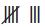?
Options:
a. 5
b. 6
c. 7
d. 8

Explanation:Question 5.
A manager at a shoe store received an order for 346 pairs of shoes. What is 346 rounded to the nearest hundred?
Options:
a. 400
b. 350
c. 340
d. 300

Explanation:
Round 346 to nearest hundred.Since 4 < 5, the digit in the rounding place stays the same. i.e, 3
Now, write zeros to the right of the rounding place (tens and ones place)
So, 346 rounded to the nearest hundred is 300.

Go Math Chapter 3 Review Test 3rd Grade Question 6.
Toby is making a picture graph. Each picture of a book is equal to 2 books he has read. The row for Month 1 has 3 pictures of books. How many books did Toby read during Month 1?
Options:
a. 2
b. 3
c. 6
d. 8

Explanation:
Given, each picture  = 2 books
Row for month 1 = 3 pictures of books = 3 x 2 = 6
So, Toby read 6 books during month 1.

### Mid-Chapter Checkpoint Page No 157

Choose the best term from the box.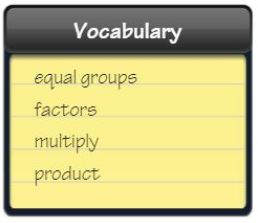Question 1.
When you combine equal groups, you can _______________ to find how many in all.
_________

Explanation:
When equal groups are combined together, multiplication operation is performed to find the total.

Question 2.
The answer in a multiplication problem is called the _________________ .
_________

Explanation:
The Product is the answer to a multiplication problem.

Question 3.
The numbers you multiply are called the ______________ .
_________

Explanation:
The numbers which are multiplied are called factors.

Concepts and Skills

Count equal groups to find how many.

Question 4._______ groups of _______ ; _______ in all

3 groups of 3; 9 in all

Explanation:
From the figure, we can say that there are 3 equal groups.
Each group has three counters.
So, the total no of counters are 9 in all.

Question 5.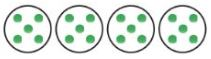_______ groups of _______ ; _______ in all

4 groups of 5; 20 in all

Explanation:
From the figure, we can say that there are 4 equal groups.
Each group has five counters.
So, the total no of counters are 20 in all.

Question 6._______ groups of _______ ; _______ in all

2 groups of 10; 20 in all

Explanation:
From the figure, we can say that there are 2 equal groups.
Each group has 10 counters.
So, the total no of counters are 20 in all.

Write related addition and multiplication sentences.

Question 7.
3 groups of 9
_______ + _______ + _______ = _______ ; _______ × _______ = _______

Answer: 9 + 9 + 9 = 27; 3 x 9 = 27

Explanation:
Draw 3 circles as groups.
Draw 9 counters in each circle.
Now, find the number of counters.
Addition Sentence: 3 groups of 9 = 9 + 9 + 9 = 27
Multiplication Sentence: 3 groups of 9 = 3 x 9 = 27

Question 8.
5 groups of 7
Type below:
________
Answer: 7 + 7 + 7 + 7 + 7 = 35; 5 x 7 = 35

Explanation:
Draw 5 circles as groups.
Draw 7 counters in each circle.
Now, find the number of counters.
Addition Sentence: 5 groups of 7 = 7 + 7 + 7 + 7 + 7 = 35
Multiplication Sentence: 5 groups of 7 = 5 x 7 = 35

Draw jumps on the number line to show equal groups.
Find the product.

Question 9.
6 groups of 3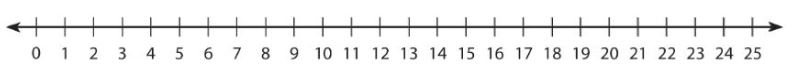_______ × _______ = _______

Answer: 6 x 3 = 18

Explanation:
Given, 6 groups of 3
No. of jumps = 6
Length of each jump = 3
Product = No. of jumps x length of each jump = 6 x 3 = 18

### Mid-Chapter Checkpoint Page No 158

Question 10.
Beth’s mother cut some melons into equal slices. She put 4 slices each on 8 plates. Write a multiplication sentence to show the total number of melon slices she put on the plates.
Type below:
_________

Answer:  8 x 4 = 32

Explanation:
Given data,
There are 8 groups which is shown as plates.
Each group has 4 counters which is shown as slices.
Since there are equal no. of slices in each plate, we can multiply to find the total number of melon slices.
Now, we can write multiplication sentences as 8 x 4 = 32.

Go Math 3rd Grade Chapter 3 Review Test Question 11.
Avery had 125 animal stickers. She gave 5 animal stickers to each of her 10 friends. How many animal stickers did she have left? What number of sentences did you use to solve?
_________ stickers left

Answer: Multiplication and Subtraction Sentence, 75

Explanation:
Given the total number of animal stickers = 125
She gave 5 animal stickers to each of her 10 friends.
Use multiplicative sentences to find no. of stickers given to her friends.
Product = 5 x 10= 50
Total no. of stickers given to her friends = 50
Now, the Total no. of stickers she left with = 125 – 50 = 75.

Question 12.
Matt made 2 equal groups of marbles. Write a multiplication sentence to show the total number of marbles.Type below:
_________

Answer: 2 x 8 = 16

Explanation:
From the above figure, we can see
There are 2 equal groups.
Each group has 8 marbles.

Question 13.
Lindsey has 10 inches of ribbon. She buys another 3 lengths of ribbon, each 5 inches long. How much ribbon does she have now?
__________ inches of ribbon

Explanation:
Given, Lindsey has 10 inches of ribbon.
She buys another 3 lengths of ribbon, each 5 inches long.
Use multiplication sentence to find length of 5 inches = 3 groups of 5
Product = 3 x 5 = 15 inches
Now, add to find how much ribbon lindsey has in all.
15 + 10 = 25
Total length of ribbon = 25 inches.

Question 14.
Jack’s birthday is in 4 weeks. How many days is it until Jack’s birthday? Describe how you could use a number line to solve
__________ days

Explanation:
Given, Jack’s birthday is in 4 weeks.
Since each week has 7 days, length of each jump = 7
Use number line to find the no. of days.
No. of jumps = 4
Now, begin at o. Skip count by 7’s by drawing jumps on the number line.
Multiply no. of jumps and length of each jump
4 x 7 = 28
So, jack’s birthday is in 28 days.

### Problem Solving Model Multiplication Page No 163

Draw a diagram to solve each problem.

Question 1.
Robert put some toy blocks into 3 rows. There are 5 blocks in each row. How many blocks are there in all?
15 blocks

Explanation:
Given, Robert put some toy blocks into 3 rows.
Each row contain 5 blocks.
Now, use bar model to find the no. of blocks in all.
Write 5 in each box to show 5 blocks in each of the 3 rows.
Since, there are equal groups, we can multiply to find the number of blocks in all.
3 x 5 = 15 blocks
So, there are 15 blocks in all.

Question 2.
Mr. Fernandez is putting tiles on his kitchen floor. There are 2 rows with 9 tiles in each row. How many tiles are there in all?
___________ tiles

Explanation:
Given, Mr. Fernandez is putting tiles on his kitchen floor.
There are 2 rows.
Each row contain 9 tiles.
Now, use bar model to find the no. of tiles in all.
Write 9 in each box to show 9 tiles in each of the 2 rows.
Since, there are equal groups, we can multiply to find the number of tiles in all.
2 x 9 = 18 tiles
So, there are 18 tiles in all.

In Jillian’s garden, there are 3 rows of carrots, 2 rows of string beans, and 1 row of peas. There are 8 plants in each row. How many plants are there in all?
___________ plants

Explanation:
Given, there are 3 rows of carrots, 2 rows of string beans, and 1 rows of peas.
Total no. of rows = 6
There are 8 plants in each row.
Now, use bar model to find the no. of plants in all.
Write 8 in each box to show 8 plants in each of the 6 rows.
Since, there are equal groups, we can multiply to find the number of plants in all.
6 x 8 = 48 plants
So, there are 48 plants in all.

Question 4.
In Sorhab’s classroom, there are 3 rows with 7 desks in each row. How many desks are there in all?
_________ desks

Explanation:
Given, there are 3 rows.
Each row contain 7 desks.
Now, use bar model to find the no. of desks in all.
Write 7 in each box to show 7 desks in each of the 3 rows.
Since, there are equal groups, we can multiply to find the number of desks in all.
3 x 7 = 21 desks
So, there are  21 desks in all.

Question 5.
Maya visits the movie rental store. On one wall, there are 6 DVDs on each of 5 shelves. On another wall, there are 4 DVDs on each of 4 shelves. How many DVDs are there in all?
___________ DVDs

Explanation:
Given, there are 2 walls.
On one wall, there are 5 shelves.
Each shelf has 6 DVDs.
Now, use bar model to find the no. of DVDs on one wall.
Write 6 in each box to show 6 DVDs on each of the 5 shelves.
Since, there are equal groups, we can multiply to find the number of DVDs on one wall.
5 x 6 = 30 DVDs
So, there are 30 DVD’s on one wall.
On another wall, there are 4 shelves.
Each shelf has 4 DVDs.
Now, use bar model to find the no. of DVDs on another wall.
Write 4 in each box to show 4 DVDs on each of the 4 shelves.
Since, there are equal groups, we can multiply to find the number of DVDs on another wall.
4 x 4 = 16 DVDs
So, there are 16 DVD’s on another wall.
Now, find the total no. of DVD’s on both all the walls.
Add. 30 + 16 = 46 DVDs.
So, there are 46 DVD’s in all.

Question 6.
The media center at Josh’s school has a computer area. The first 4 rows have 6 computers each. The fifth row has 4 computers. How many computers are there in all?
___________ computers

Explanation:
Given, there are 4 rows.
Each row contain 6 computers.
Now, use bar model to find the no. of computers in all.
Write 6 in each box to show 6 computers in each of the 4 rows.
Since, there are equal groups, we can multiply to find the number of computers in all.
4 x 6 = 24 computers
So, there are 24 computers in all.
There is another fifth row with 4 computers.
Now, use bar model to find the no. of computers in all.
No. of computers in fifth row = 4 x 1 = 4 computers.
Add the computers in all rows to find how many in all.
24 + 4 = 28
So, there are 28 computers in all.

### Model Multiplication Lesson Check Page No 164

Question 1.
There are 5 shelves of video games in a video store. There are 6 video games on each shelf. How many video games are there in all?
Options:
a. 35
b. 30
c. 20
d. 11

Explanation:
Given, there are 5 shelves.
Each shelf contain 6 video games.
Now, use bar model to find the no. of video games in all.
Write 6 in each box to show 6 video games in each of the 5 shelves.
Since, there are equal groups, we can multiply to find the number of shelves in all.
5 x 6 = 30 shelves
So, there are 30 shelves in all.

3rd Grade Go Math Chapter 3 Test Question 2.
Ken watches a marching band. He sees 2 rows of flute players. Six people are in each row. He sees 8 trombone players. How many flute or trombone players does Ken see?
Options:
a. 2
b. 6
c. 16
d. 20

Explanation:
Given, there are 6 flute players in each row.
There are 2 rows.
Now, use bar model to find the no. of flute players in all.
Write 6 in each box to show 6 flute players in each of the 2 rows.
Since, there are equal groups, we can multiply to find the number of flute players in all.
2 x 6 = 12 flute players
Given, there are 8 trombone players.
Now, add flute and trombone players
12 + 8 = 20
So, there are 20 flute or trombone players.

Spiral Review

Question 3.
What is the sum of 438 and 382?
Options:
a. 720
b. 810
c. 820
d. 910

Explanation:
Step 1:
Add ones. Regroup ones as tens and ones.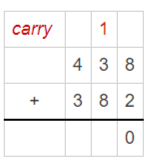Step 2:
Add tens. Regroup tens as hundreds and tens.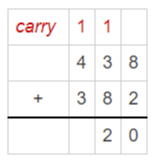Step 3: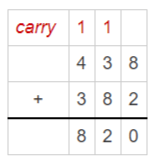Question 4.
Estimate the sum.
6 2 2
+ 8 4
———
Options:
a. 500
b. 600
c. 700
d. 800

Explanation:
Use compatible numbers.
622 —-> 600
84  —–> 100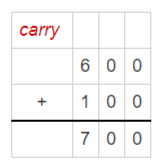Question 5.
Francine uses 167 silver balloons and 182 gold balloons for her store party. How many silver and gold balloons in all does Francine use?
Options:
a. 15
b. 345
c. 349
d. 359

Explanation:
Given, Silver balloons = 167
Gold balloons = 182
Add Silver and gold balloons to find total in all.Step 2: Add tens. Regroup tens as hundreds and tens.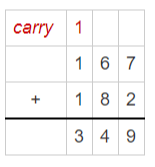So, total no. of silver and gold balloons in all does Francine use = 349

Question 6.
Yoshi is making a picture graph. Each picture of a soccer ball stands for two goals he scored for his team. The row for January has 9 soccer balls. How many goals did Yoshi score during January?
Options:
a. 18
b. 16
c. 11
d. 9

Explanation:
Given, each picture = 2 goals
Row for January = 9 soccer balls
Consider each picture as 9 soccer balls.
Now, find the goals did Yoshi score during January.
9 x 2 = 18
So, 18 goals did Yoshi score during January.

### Model with Arrays Page No 169

Write a multiplication sentence for the array.

Question 1.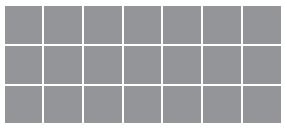3 × 7 = 21

Answer: 3 x 7 = 21

Explanation:
In the above figure, we can see there are same no. of tiles in each row.
There are 3 rows with 7 tiles in each row.
Now, multiplication sentence for array can be written as number of rows x no. of tiles in each row.
Multiply. 3 x 7 = 21

Question 2.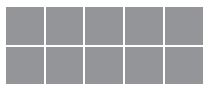2 × 5 = _______

Explanation:
In the above figure, we can see there are same no. of tiles in each row.
There are 2 rows with 5 tiles in each row.
Now, multiplication sentence for array can be written as number of rows x no. of tiles in each row.
Multiply. 2 x 5 = 10

Draw an array to find the product.

Question 3.
4 × 2 = _______

Explanation:
Make an array by placing the same no. of tiles in each row.
From the given data, make an array with 4 rows of 2 tiles.
Now, draw an array.
In the above figure, we can see there are same no. of tiles in each row.
There are 4 rows with 2 tiles in each row.
Now, multiplication sentence for array can be written as number of rows x no. of tiles in each row.
Multiply. 4 x 2 = 8

Question 4.
4 × 4 = _______

Explanation:
Make an array by placing the same no. of tiles in each row.
From the given data, make an array with 4 rows of 4 tiles.
Now, draw an array.
In the above figure, we can see there are same no. of tiles in each row.
There are 4 rows with 4 tiles in each row.
Now, multiplication sentence for array can be written as number of rows x no. of tiles in each row.
Multiply. 4 x 4 = 16

Question 5.
3 × 2 = _________

Explanation:
Make an array by placing the same no. of tiles in each row.
From the given data, make an array with 3 rows of 2 tiles.
Now, draw an array.
In the above figure, we can see there are same no. of tiles in each row.
There are 3 rows with 2 tiles in each row.
Now, multiplication sentence for array can be written as number of rows x no. of tiles in each row.
Multiply. 3 x 2 = 6

Question 6.
2 × 8 = _______

Explanation:
Make an array by placing the same no. of tiles in each row.
From the given data, make an array with 2 rows of 8 tiles.
Now, draw an array.
In the above figure, we can see there are same no. of tiles in each row.
There are 2 rows with 8 tiles in each row.
Now, multiplication sentence for array can be written as number of rows x no. of tiles in each row.
Multiply. 2 x 8 = 16

Problem Solving

Question 7.
Lenny is moving tables in the school cafeteria. He places all the tables in a 7 × 4 array. How many tables are in the cafeteria?
_________ tables

Explanation:
Given array = 7 x 4
Now, make an array with 7 rows of 4 tiles.
Draw an array and find the no. of tables.
Multiply. 7 x 4 = 28
So, there are 28 tables in the cafeteria.

Question 8.
Ms. DiMeo directs the school choir. She has the singers stand in 3 rows. There are 8 singers in each row. How many singers are there in all?
_________ singers

Explanation:
Given, no. of singers in each row = 8
No. of rows = 3
Now, make an array with 3 rows of 8 singers.
Find the no. of singers by multiplying no. of rows with singers.
Multiply. 3 x 8 = 24 singers.
So, there are 24 singers in all.

### Model with Arrays Lesson Check Page No 170

Question 1.
What multiplication sentence does this array show?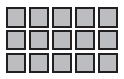Options:
a. 2 × 3 = 6
b. 6 × 3 = 18
c. 3 × 4 = 12
d. 3 × 5 = 15

Explanation:
From the above figure, we can see that the array consists of 3 rows and 5 tiles.
Now, the multiplications sentence is 3 x 5 = 15

Question 2.
What multiplication sentence does this array show?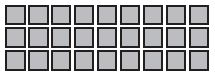Options:
a. 3 × 9 = 27
b. 3 × 8 = 24
c. 3 × 7 = 21
d. 4 × 5 = 20

Explanation:
From the above figure, we can see that the array consists of 3 rows and 9 tiles.
Now, the multiplications sentence is 3 x 9 = 27

Spiral Review

Question 3.
Use the table to find who traveled 700 miles farther than Paul during summer vacation.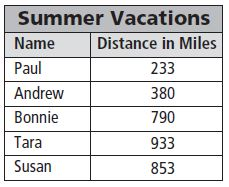Options:
a. Andrew
b. Bonnie
c. Susan
d. Tara

Explanation:
From the table, we can say that paul travelled 233 miles.
Now, use break apart strategy to find sums.
Paul –>      233 = 200 + 30 + 3
Andrew –> 380 = 300 + 80+ 0
Bonnie –>  790 = 700 + 90+ 0
Tara –>      933 = 900 + 30+ 3
Susan –>   853 = 800 + 50+ 3
From the above sums, we can say that Tara travelled farther miles than Paul.

Use the bar graph to find what hair color most students have.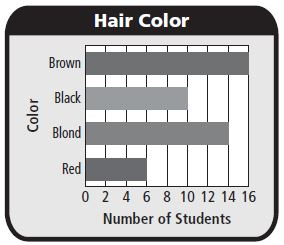Options:
a. Brown
b. Black
c. Blond
d. Red

Explanation:
The title shows hair color for students.
The length of bar tells the no. of students had each color.
Now, Find out the which color maximum no. of students have.
We can see that the brown color is the highest.

Question 5.
Spencer ordered 235 cans of tomatoes to make salsa for the festival. What is 235 rounded to the nearest ten?
Options:
a. 200
b. 230
c. 240
d. 300

Explanation:
Round 235 to nearest ten.
The digit to the right of rounding place is 5.
So, the digit in the rounding place is increased by one.
Write zero to the round of rounding digit.
Now, it becomes 240.

Question 6.
Which bar would be the longest on a bar graph of the data?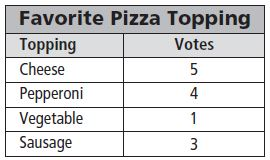Options:
a. Cheese
b. Pepperoni
c. Vegetable
d. Sausage

Explanation:
Make a bar graph.
Step 1:
Write a title at the top to tell what the graph is about. Label the side of the graph to tell about the bars. Label the bottom of the graph to explain what the numbers tell.
Step 2:
Choose numbers for the bottom of the graph so that most of the bars will end on a line. Since the least number is 1 and the greatest number is 5, make the scale 0-5.
Step 3: Draw and shade a bar to show the number for each pizza topping.
From the bar graph, we can say that the cheese bar would be the longest on a bar graph of the data.

### Commutative Property of Multiplication Page No 175

Write a multiplication sentence for the model. Then use the Commutative Property of Multiplication to write a related multiplication sentence.

Question 1.5 × 2 = 10
2 × 5 = 10

Answer: 5 × 2 = 10
2 × 5 = 10

Explanation:
From the figure, we can say that there are 5 rows.
There are two tiles in each row.
From the given array, the multiplication sentence can be written as 5 x 2 = 10
The Commutative property of multiplication have the same factors in different order.
So, by using commutative property, Multiplication sentence is written as 2 x 5 = 10.

Question 2.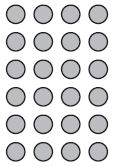______ × _____ = _______
______ × _____ = _______

6 x 4 = 24
4 x 6 = 24

Explanation:
From the figure, we can say that there are 6 rows.
There are 4 tiles in each row.
From the given array, the multiplication sentence can be written as 6 x 4 = 24
The Commutative property of multiplication have the same factors in different order.
So, by using commutative property, Multiplication sentence is written as 4 x 6 = 24.

Question 3.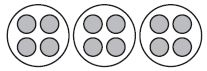______ × _____ = _______
______ × _____ = _______

3 x 4 = 12
4 x 3 = 12

Explanation:
From the figure, we can say that there are 3 equal groups.
There are 4 counters in each group.
From the given array, the multiplication sentence can be written as 3 x 4 = 12
The Commutative property of multiplication have the same factors in different order.
So, by using commutative property, Multiplication sentence is written as 4 x 3 = 12.

Question 4.______ × _____ = _______
______ × _____ = _______

2 x 6 = 12
6 x 2 = 12

Explanation:
From the figure, we can say that there are 2 equal groups.
There are 6 counters in each group.
From the given array, the multiplication sentence can be written as 2 x 6 = 12
The Commutative property of multiplication have the same factors in different order.
So, by using commutative property, Multiplication sentence is written as 6 x 2 = 12.

Problem Solving

Question 5.
A garden store sells trays of plants. Each tray holds 2 rows of 8 plants. How many plants are in one tray?
___________ plants

16 plants

Explanation:
Given,there are 8 plants.
Each tray holds 2 rows of 8 plants.
Now, by using multiplicative sentence or commutative property, we can find the no. of plants in one tray.
Multiplicative Sentence : 2 x 8 = 16 plants
Commutative property of multiplication: 8 x 2 = 16
So, there are 16 plants in one tray.

Question 6.
Jeff collects toy cars. They are displayed in a case that has 4 rows. There are 6 cars in each row. How many cars does Jeff have?
________ cars

24 cars

Explanation:
Given, toy cars are displayed in 4 rows.
There are 6 cars in each row.
Now, by using multiplicative sentences or commutative property, we can find the no. of cars in each row.
Multiplicative Sentence : 6 x 4 = 24 cars.
Commutative property of multiplication: 4 x 6 = 24 cars.

### Commutative Property of Multiplication Lesson Check Page No 176

Question 1.
Which is an example of the Commutative Property of Multiplication?
Options:
a. 8 × 4 = 8 × 4
b. 4 × 2 = 2 × 4
c. 2 × 8 = 4 × 4
d. 2 + 4 = 2 × 4

Explanation:
The Commutative Property of Multiplication states that when you change the order
of the factors, the product stays the same. From the given options, 4 x 2 = 2 x 4 is an example of commutative property of multiplication.

Question 2.
What factor makes the number sentence true?
7 × 4 = ■ × 7
Options:
a. 2
b. 4
c. 7
d. 28

Explanation:
The Commutative Property of Multiplication states that when you change the order
of the factors, the product stays the same. From the question, we can see that 7 x 4 = 28;
Then 4 x 7 = 28

Spiral Review

Question 3.
Ms. Williams drove 149 miles on Thursday and 159 miles on Friday. About how many miles did she drive altogether the two days?
Options:

Explanation:

Given, miles driven by Ms. Williams on Thursday = 149
Miles driven by Ms. Williams on Friday = 159
By using compatible numbers, we can find the no. of miles did she drive altogether the two days.
149 —> 150
159 —> 150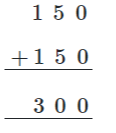So, the no. of miles driven altogether is about 300 miles.

Question 4.
Inez has 699 pennies and 198 nickels. Estimate how many more pennies than nickels she has.
Options:

Explanation:

Given, there are 699 pennies and 198 nickels.
Now, estimate to find how many more pennies than nickels she has.
Use compatible numbers to compute it.
699 —> 700
198 —> 200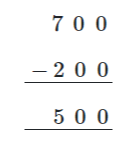So, Inez has 500 more pennies than nickels.

Question 5.
This year, the parade had 127 floats. That is 34 fewer floats than last year. How many floats were in the parade last year?
Options:
a. 161
b. 151
c. 103
d. 93

Explanation:
Given, the no. of floats this year = 127
No. of floats last year is 34 greater than this year.
Now, estimate the no. of floats in the parade last year
Step 1: Add ones. Regroup the ones as tens and ones.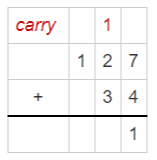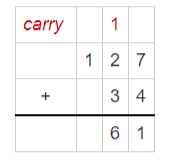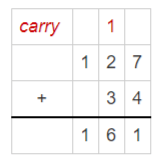The total no. of floats in the parade last year = 161

Question 6.
Jeremy made a tally table to record how his friends voted for their favorite pet. His table showsnext to Dog. How many friends voted for dog?
Options:
a. 6
b. 8
c. 10
d. 12

Explanation:
Count the tally marks. It shows 12. So, the no. of friends voted for dog = 12

### Multiply with 1 and 0 Page No 181

Find the product.

Question 1.
1 × 4 = 4

Explanation:
The Identity Property of Multiplication states that the product of any number and 1 is that number.
So, 1 x 4 = 4

Question 2.
0 × 8 = _______

Explanation:
The Zero Property of Multiplication states that the product of zero and any number is zero
So, 0 x 8 = 0

Question 3.
0 × 4 = _______

Explanation:
The Zero Property of Multiplication states that the product of zero and any number is zero.
So, 0 x 4 = 0

Question 4.
1 × 6 = _______

Explanation:
The Identity Property of Multiplication states that the product of any number and 1 is that number.
So, 1 x 6 = 6

Question 5.
3 × 0 = _______

The Zero Property of Multiplication states that the product of zero and any number is zero.
So, 3 x 0 = 0

Question 6.
0 × 9 = _______

The Zero Property of Multiplication states that the product of zero and any number is zero.
So, 0 x 9 = 0

Question 7.
8 × 1 = _______

Explanation:
The Identity Property of Multiplication states that the product of any number and 1 is that number.
So, 8 x 1 = 6

Question 8.
1 × 2 = _______

Explanation:
The Identity Property of Multiplication states that the product of any number and 1 is that number.
So, 1 x 2 = 2

Question 9.
0 × 6 = _______

The Zero Property of Multiplication states that the product of zero and any number is zero.
So, 0 x 6 = 0

Question 10.
4 × 0 = _______

The Zero Property of Multiplication states that the product of zero and any number is zero.
So, 4 x 0 = 0

Question 11.
7 × 1 = _______

Explanation:
The Identity Property of Multiplication states that the product of any number and 1 is that number.
So, 7 x 1 = 7

Question 12.
1 × 5 = _______

Explanation:
The Identity Property of Multiplication states that the product of any number and 1 is that number.
So, 1 x 5 = 5

Question 13.
3 × 1 = _______

Explanation:
The Identity Property of Multiplication states that the product of any number and 1 is that number.
So, 3 x 1 = 3

Question 14.
0 × 7 = _______

The Zero Property of Multiplication states that the product of zero and any number is zero.
So, 0 x 7 = 0

Question 15.
1 × 9 = _______

Explanation:
The Identity Property of Multiplication states that the product of any number and 1 is that number.
So, 1 x 9 = 9

Question 16.
5 × 0 = _______

The Zero Property of Multiplication states that the product of zero and any number is zero.
So, 5 x 0 = 0

Question 17.
10 × 1 = _______

Explanation:
The Identity Property of Multiplication states that the product of any number and 1 is that number.
So, 10 x 1 = 10

Question 18.
2 × 0 = _______

The Zero Property of Multiplication states that the product of zero and any number is zero.
So, 2 x 0 = 0

Question 19.
5 × 1 = _______

Explanation:
The Identity Property of Multiplication states that the product of any number and 1 is that number.
So, 5 x 1 = 5

Question 20.
1 × 0 = _______

Explanation:
The Zero Property of Multiplication states that the product of zero and any number is zero.
So, 1 x 0 = 0

Question 21.
0 × 0 = _______

Explanation:
The Zero Property of Multiplication states that the product of zero and any number is zero.
So, 1 x 0 = 0

Question 22.
1 × 3 = _______

Explanation:
The Identity Property of Multiplication states that the product of any number and 1 is that number.
So, 1 x 3 = 3

Question 23.
9 × 0 = _______

Explanation:
The Zero Property of Multiplication states that the product of zero and any number is zero.
So, 9 x 0 = 0

Question 24.
1 × 1 = _______

Explanation:
The Identity Property of Multiplication states that the product of any number and 1 is that number.
So, 1 x 1 = 1

Problem Solving

Question 25.
Peter is in the school play. His teacher gave 1 copy of the play to each of 6 students. How many copies of the play did the teacher hand out?
_________ copy

Explanation:
Given, No. of students = 6
Copies given to each student = 1
No. of copies of the play did teacher hand out = 1 x 6 = 6

Question 26.
There are 4 egg cartons on the table. There are 0 eggs in each carton. How many eggs are there in all?
_________ eggs

Explanation:
Given, there are 4 egg cartons
There are 0 eggs in each carton
No. of eggs in all = ?
0 x 4 = 0 eggs.
No. of eggs in all = 0

### Multiply with 1 and 0 Lesson Check Page No 182

Question 1.
There are 0 bicycles in each bicycle rack. If there are 8 bicycle racks, how many bicycles are there in all?
Options:
a. 80
b. 8
c. 1
d. 0

Explanation:
There are 0 bicycles in each bicycle rack.
There are 8 bicycle racks.
No. of bicycles in all = 8 x 0 = 0

Question 2.
What is the product?
1 × 0 = _______
Options:
a. 0
b. 1
c. 10
d. 11

Explanation:
The Zero Property of Multiplication states that the product of zero and any number is zero.
So, 1 x 0 = 0

Spiral Review

Question 3.
Mr. Ellis drove 197 miles on Monday and 168 miles on Tuesday. How many miles did he drive in all?
Options:
a. 29 miles
b. 255 miles
c. 365 miles
d. 400 miles

Explanation:
Given, no. of miles driven on Monday = 197
no. of miles driven on Tuesday = 168
Perform addition to find the no. of miles driven in all

Question 4.
What multiplication sentence does the array show?
■ ■ ■ ■ ■ ■
Options:
a. 1 × 6 = 6
b. 3 × 2 = 6
c. 2 × 6 = 12
d. 5 + 1 = 6

Explanation:
The given array shows 1 row with 6 tiles.
So, a multiplication sentence can be written as 1 x 6 = 6

Use the bar graph for 5–6.Question 5.
How many cars were washed on Friday and Saturday combined?
Options:
a. 55
b. 80
c. 90
d. 120

Explanation:
From the bar graph, we can see that no. of cars washed on Friday = 25
No. of cars washed on Saturday = 55
Total no. of cars washed on Friday and Saturday = 80

Question 6.
How many more cars were washed on Saturday than on Sunday?
Options:
a. 95
b. 30
c. 25
d. 15

Explanation:
From the bar graph, we can see that cars washed on Saturday = 55
Cars washed on Sunday = 40
55-40 = 15
15 more cars were washed on Saturday than on Sunday.

### Chapter 3 Review Test Page No 183

Question 1.
There are 3 boats on the lake. Six people ride in each boat. How many people ride in the boats? Draw circles to model the problem and explain how to solve it.
_________ people

Explanation:
Given, no. of boats on the lake = 3
No. of people ride in each boat = 6
Total no. of people ride in the boats = 3 groups of 6
3 x 6 = 18
So, 18 people ride in the boats.

Question 2.
Nadia has 4 sheets of stickers. There are 8 stickers on each sheet. She wrote this number sentence to represent the total number of stickers.

4 × 8 = 32

What is a related number sentence that also represents the total number of stickers she has?
Options:
a. 8 + 4 =■
b. 4 + 4 + 4 + 4 = ■
c. 8 × 8 = ■
d. 8 × 4 = ■

Explanation:
Given, there are 4 sheets of stickers.
Each sheet has 8 stickers.
Given total no. of stickers represented as = 4 x 8 = 32
By using the commutative property of multiplication, a related number sentence can be represented as 8 x 4 = 32

Lindsay went hiking for two days in Yellowstone National Park. The first jump on the number line shows how many birds she saw on the first day. She saw the same number of birds the next day.Write the multiplication sentence that is shown on the number line.
______ × _______ = _______

Answer: 2 x 8 = 16

Explanation:
Given, the total no. of jumps = 2
From the figure, we can see that there are two jumps which begins at 0 and skip count by 8’s.
Product = No. of jumps x Length of each jump.
Now, multiplication sentence can be written as 2 x 8 = 16

### Chapter 3 Review Test Page No 184

Question 4.
Paco drew an array to show the number of desks in his classroom. Write a multiplication sentence for the array.________ desks

Explanation:
In the given array, there are three rows.
Each row has 7 desks.
Now, multiplication sentence can be written as no. of rows x no. of desks in each row.
Multiply. 3 x 7 = 21 desks

Question 5.
Alondra makes 4 necklaces. She uses 5 beads on each necklace. For numbers 5a–5d, choose Yes or No to tell if the number sentence could be used to find the number of beads Alondra uses.
a. 4 × 5 = ■
i. yes
ii. no

Explanation: Given, there are 4 necklaces.
Now, number sentence (multiplication) can be written as 4 groups of 5 = 4 x 5

Question 5.
b. 4 + 4 + 4 + 4 = ■
i. yes
ii. no

Given, there are 4 necklaces.
Now, number sentence (addition) can be written as 4 groups of 5 = 5 + 5 + 5 + 5

Question 5.
c. 5 + 5 + 5 + 5 = ■
i. yes
ii. no

Given, Given, there are 4 necklaces.
Now, number sentence (addition) can be written as 4 groups of 5 = 5 + 5 + 5 + 5

Question 5.
d. 5 + 4 = ■
i. yes
ii. no

Given, there are 4 necklaces.
Now, number sentence (addition) can be written as 4 groups of 5 = 4 x 5
Using commutative property of multiplication, it can be written as 5 x 4, but given question is 5 + 4.

Question 6.
John sold 3 baskets of apples at the market. Each basket contained 9 apples. How many apples did John sell? Make a bar model to solve the problem._______ apples

Explanation:
Given, there are 3 baskets of apples.
Draw a bar model with 3 boxes to show 3 baskets.
Write 9 in each box to show 9 apples.
Since, there are equal groups, we can multiply to find No. of apples john sold.
3 x 9 = 27 apples.

### Chapter 3 Review Test Page No 185

Question 7.
Select the number sentences that show the Commutative Property of Multiplication. Mark all that apply.
Options:
a. 3 × 2 = 2 × 3
b. 4 × 9 = 4 × 9
c. 5 × 0 = 0
d. 6 × 1 = 1 × 6
e. 7 × 2 = 14 × 1

Explanation:
Commutative Property of Multiplication states that when you change the order of the factors, the product stays the same.
So, 3 x 2 = 2 x 3 is the answer.

Question 8.
A waiter carried 6 baskets with 5 dinner rolls in each basket. How many dinner rolls did he carry? Show your work.
___________ dinner rolls

Explanation:
Each basket has 5 dinner rolls.
No. of dinner rolls = 6 x 5 = 30 dinner rolls.

Question 9.
Sonya needs 3 equal lengths of wire to make 3 bracelets. The jump on the number line shows the length of one wire in inches. How many inches of wire will Sonya need to make the 3 bracelets?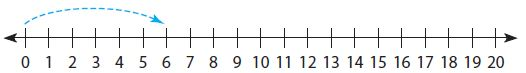_________ inches

Explanation:
Given, Length of one wire = jump on the number line
From the figure, we can see that length of wire = 6 inches
3 equal lengths of wire is required for 3 bracelets.
So, no. of jumps = 3
length of each jump = 6 inches
Multiply. 3 x 6 = 18
So, sonya need 18 inches of wire to make 3 bracelets.

Question 10.
Josh has 4 dogs. Each dog gets 2 dog biscuits every day. How many biscuits will Josh need for all of his dogs for Saturday and Sunday?
__________ biscuits

Explanation:
Given, there are 4 dogs.
Each dog gets 2 biscuits every day.
No. of biscuits required for all dogs every day = 4 x 2 = 8
No. of biscuits need for all dogs for Saturday and Sunday = 8 x 2 = 16 biscuits.

### Chapter 3 Review Test Page No 186

Question 11.
Jorge displayed 28 cans of paint on a shelf in his store.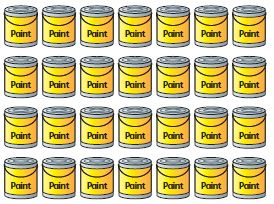Select other ways Jorge could arrange the same number of cans. Mark all that apply.
Options:
a. 2 rows of 14
b. 1 row of 28
c. 6 rows of 5
d. 8 rows of 3
e. 7 rows of 4

Explanation:
Given, There are 28 cans of paint on a shelf in a store.
In the given array, there are 4 rows with 7 cans in each row.
So, there are 4 rows of 7.
By using commutative property of multiplication, it can be arranged in 7 rows of 4.

Question 12.Choose the number that makes the statement true. The product of any number and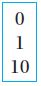is zero.

Explanation:
The Zero Property of Multiplication states that the product of any number and zero is zero.

Question 13.
James made this array to show that 3 × 5 = 15.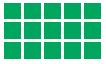Part A
James says that 5 × 3 = 15. Is James correct? Draw an array to explain your answer.
a. yes
b. no

Explanation: Draw an array to show 5 rows with 3 tiles in each row.
Then, Multiplication sentence can be written as no. of rows x no. of tiles in each row
5 x 3 = 15

Question 13.
Part B
________

Explanation:
Commutative Property of Multiplication supports this answer. Because, it states that the product of any tow factors in reverse order remains the same.

### Chapter 3 Review Test Page No 187

Question 14.
Julio has a collection of coins. He puts the coins in 2 equal groups. There are 6 coins in each group. How many coins does Julio have? Use the number line to show your work.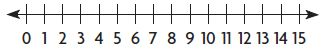________ coins

Explanation:
There are 2 equal groups.
There are 6 coins in each group.
Find total no. of coins by using number line.
Begin at 0. Skip count by 6s by drawing jumps on the number line.
So, no. of jumps = 2
Length of each jump = 6
Multiply. 2 x 6 = 12
Total no. of coins Julio have = 12

Question 15.
Part A
Yesterday, Landon sorted his trading cards into 4 groups. Each group had 7 cards. Draw a bar model to show Landon’s cards. How many cards does he have?

Explanation:
No. of groups = 4
Each group has 7 cards.
Draw a bar model to show the cards.
Draw 4 boxes in the bar model.
Write 7 in each box to show 7 cards.
Since, there are equal groups, multiply to find the no. of cards.
Multiply. 4 x 7 = 28 cards.

Question 15.
Part B
Landon buys 3 more packs of trading cards today. Each pack has 8 cards. Write a multiplication sentence to show how many cards Landon buys today. Then find how many cards Landon has now. Show your work.
Type below:
_________

Explanation:
Each pack has 8 cards.
Multiplication Sentence = 3 x 8 = 24.
Total no. of cards = 28 + 24 = 52 cards.

Question 16.
A unicycle has only 1 wheel. Write a multiplication sentence to show how many wheels there are on 9 unicycles.
_______ × _______ = _______

Answer: 9 x 1 = 9

Explanation:
Given, there are 9 unicycles.
Each unicycle has only one wheel.
So, Multiplication Sentence can be written as 9 x 1 = 9.

Question 17.
Carlos spent 5 minutes working on each of 8 math problems. He can use 8 × 5 to find the total amount of time he spent on the problems.
For numbers 17a–17d, choose Yes or No to show which are equal to 8 × 5.
a. 8 + 5
i. yes
ii. no

Explanation:
Given, Carlos spent 5 minutes working on each of 8 math problems.
Given Sentence = 8 x 5
As there are 8 problems with 5 minutes spent on each, the total time can be find out using multiplication sentence.

Question 17.
b. 5 + 5 + 5 + 5 + 5
i. yes
ii. no

Given, Carlos spent 5 minutes working on each of 8 math problems.
Given Sentence = 8 x 5 = 40
But given option 5 + 5 + 5 + 5 + 5 = 25.

Question 17.
c. 8 + 8 + 8 + 8 + 8
i. yes
ii. no

Explanation:
Given, Carlos spent 5 minutes working on each of 8 math problems.
Given Sentence = 8 x 5 = 40
Given option = 8 + 8 + 8 + 8 + 8 = 40

Question 17.
d. 5 + 5 + 5 + 5 + 5 + 5 + 5 + 5
i. yes
ii. no

Explanation:
Given, Carlos spent 5 minutes working on each of 8 math problems.
Given Sentence = 8 x 5 = 40
Given option = 5 + 5 + 5 + 5 + 5 + 5 + 5 + 5 = 40

### Chapter 3 Review Test Page No 188

Question 18.
Lucy and her mother made tacos. They put 2 tacos on each of 7 plates.
Select the number sentences that show all the tacos Lucy and her mother made. Mark all that apply.
Options:
A. 2 + 2 + 2 + 2 + 2 + 2 + 2 = 14
B. 2 + 7 = 9
C. 7 + 7 = 14
D. 8 + 6 = 14
E. 2 × 7 = 14

Explanation:
Total no. of tacos = 2
Each taco has 7 plates.
Addition Sentence can be written as 2 + 2 + 2 + 2 + 2 + 2 + 2 = 14
Multiplication Sentence can be written as 2 x 7 = 14

Question 19.
Jayson is making 5 sock puppets. He glues 2 buttons on each puppet for its eyes. He glues 1 pompom on each puppet for its nose.
Part A
Write the total number of buttons and pompoms he uses. Write a multiplication sentence for each.
Eyes, Noses
_________ buttons _________ pompoms

10 buttons,
5 x 2 = 10

Noses
5 pompoms
5 x 1 = 5

Explanation:
Given, there are 5 sock puppets
Each puppet has 2 buttons for its eyes
Each puppet has 1 pompom for its nose
Total no. of buttons
Multiplication Sentence = 5 x 2 = 10
Total no. of pompoms
Multiplication Sentence = 5 x 1 = 5

Question 19.
Part B
After making 5 puppets, Jayson has 4 buttons and 3 pompoms left. What is the greatest number of puppets he can make with those items if he wants all his puppets to look the same? Draw models and use them to explain.
_________ puppets